# RD Sharma Solutions for Class 12 Maths Exercise 18.5 Chapter 18 Maxima and Minima

RD Sharma Solutions for Class 12 Maths Exercise 18.5 Chapter 18 Maxima and Minima is available here. The solutions are designed by subject matter experts based on the grasping abilities of students. Students can make use of the solutions PDF while solving exercise-wise problems, also understanding the other possible ways of obtaining answers easily.

The PDF of RD Sharma Solutions for Class 12 Maths Chapter 18 Maxima and Minima Exercise 18.5 is available here. Students who aim to clear the exams with good marks can download the PDF from the given links.

## RD Sharma Solutions for Class 12 Chapter 18 – Maxima and Minima Exercise 18.5

### Access other exercises of RD Sharma Solutions For Class 12 Chapter 18 – Maxima and Minima

Exercise 18.1 Solutions

Exercise 18.2 Solutions

Exercise 18.3 Solutions

Exercise 18.4 Solutions

### Exercise 18.5 Page No: 18.72

1. Determine two positive numbers whose sum is 15 and the sum of whose squares is minimum.

Solution: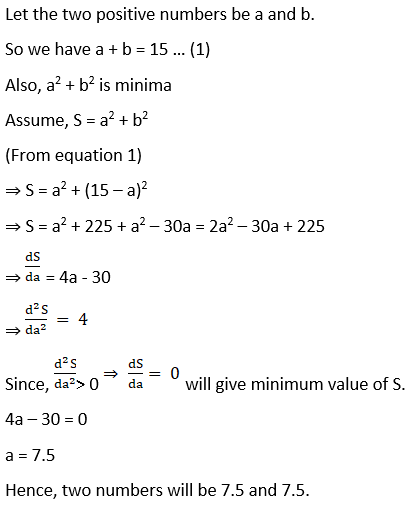Which implies S is minimum when a = 15/2 and b = 15/2.

2. Divide 64 into two parts such that the sum of the cubes of two parts is minimum.

Solution:

Let the two positive numbers be a and b.

Given a + b = 64 … (1)

We have, a3 + b3 is minima

Assume, S = a3 + b3

(From equation 1)

S = a3 + (64 – a)3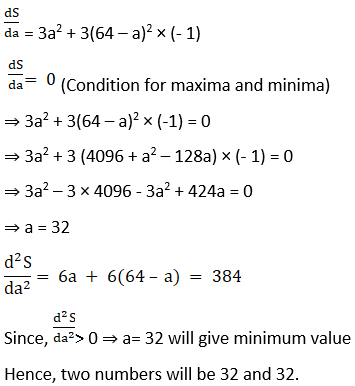of S

Hence, the two number will be 32 and 32.

3. How should we choose two numbers, each greater than or equal to –2, whose sum is ½ so that the sum of the first and the cube of the second is minimum?

Solution: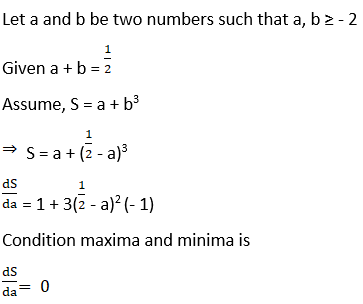dS/da = 0

1 + 3(½ – a)2 (-1) = 0

1 – 3(½ – a)2 = 0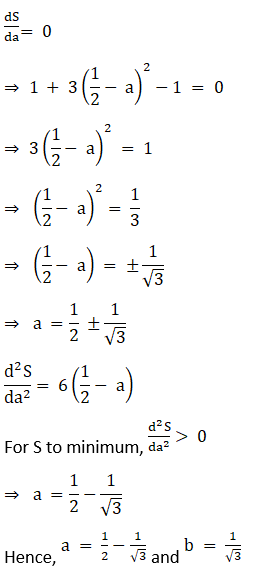4. Divide 15 into two parts such that the square of one multiplied with the cube of the other is minimum.

Solution:

Let the given two numbers be x and y. Then,

x + y = 15 ….. (1)

y = (15 – x)

Now we have z = x2 y3

z = x2 (15 – x)3 (from equation 1)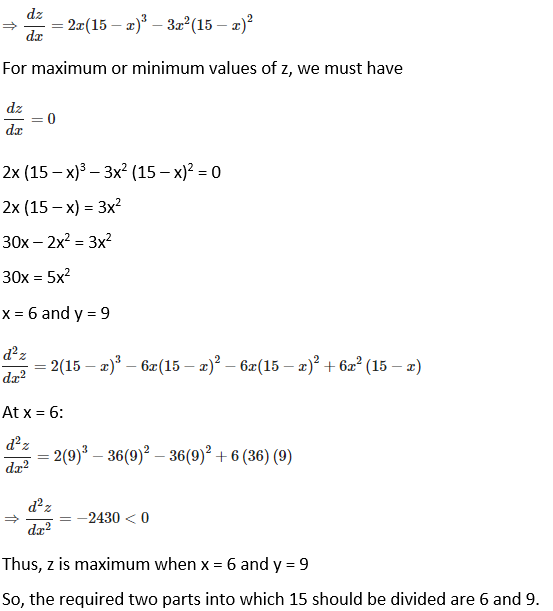5. Of all the closed cylindrical cans (right circular), which enclose a given volume of 100 cm3, which has the minimum surface area?

Solution:

Let r and h be the radius and height of the cylinder, respectively. Then,

Volume (V) of the cylinder = πr2 h

⟹ 100 = πr2 h

⟹ h = 100/ πr2

Surface area (S) of the cylinder = 2 πr2 + 2 πr h = 2 πr2 + 2 πr × 100/ πr2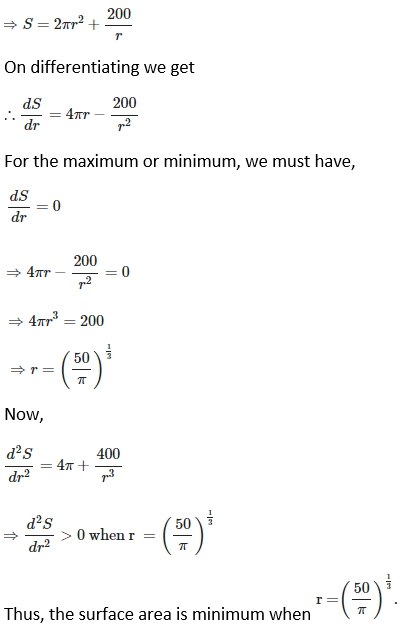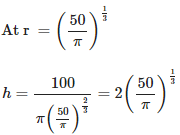6. A beam is supported at the two ends and is uniformly loaded. The bending moment M at a distance x from one end is given by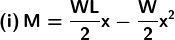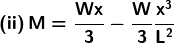Find the point at which M is maximum in each case.

Solution: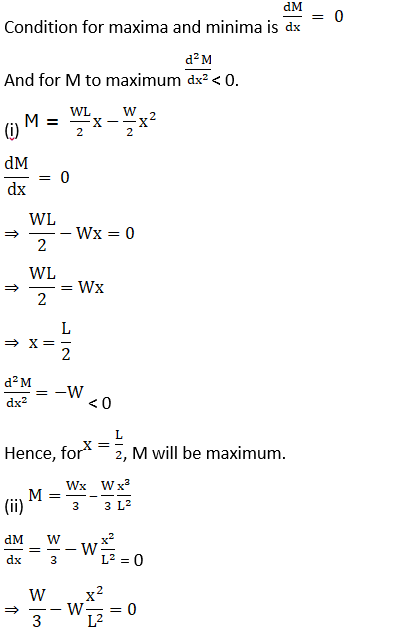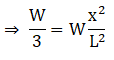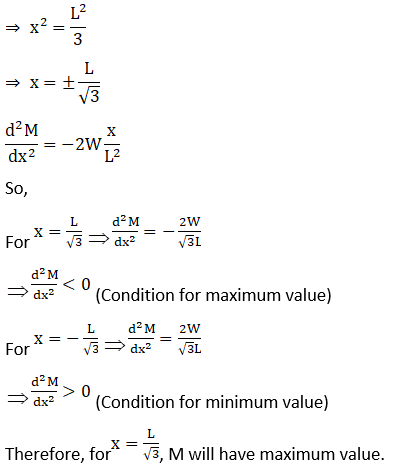7. A wire of length 28 m is to be cut into two pieces. One of the pieces is to be made into a square and the other into a circle. What should be the lengths of the two pieces so that the combined area of the circle and the square is minimum?

Solution:

Suppose the given wire, which is to be made into a square and a circle, is cut into two pieces of length x and y m respectively. Then,

x + y = 28 ⇒ y = (28 – x)

We know that the perimeter of a square, 4 (side) = x

Side = x/4

Area of square = (x/4)2 = x2/16

Circumference of a circle, 2 π r = y

r = y/ 2 π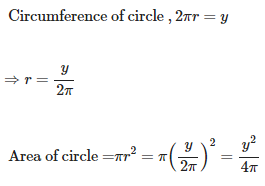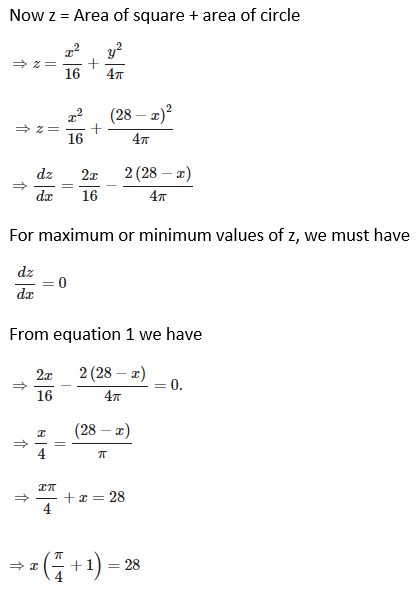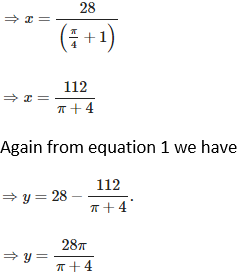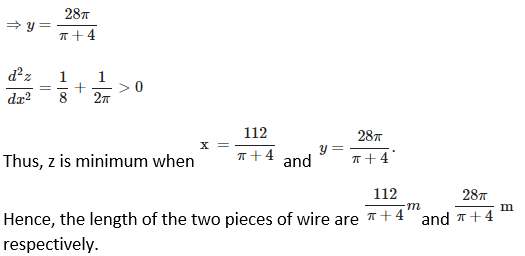8. A wire of length 20 m is to be cut into two pieces. One of the pieces will be bent into shape of a square and the other into shape of an equilateral triangle. Where the wire should be cut so that the sum of the areas of the square and triangle is minimum?

Solution:

Suppose the wire, which is to be made into a square and a triangle, is cut into two pieces of length x and y respectively. Then,

x + y = 20 ⇒ y = (20 – x) …… (1)

We know that the perimeter of a square, 4 (side) = x

Side = x/4

Area of square = (x/4)2 = x2/16

Again we know that the perimeter of a triangle, 3 (side) = y.

Side = y/3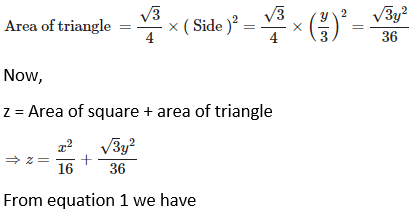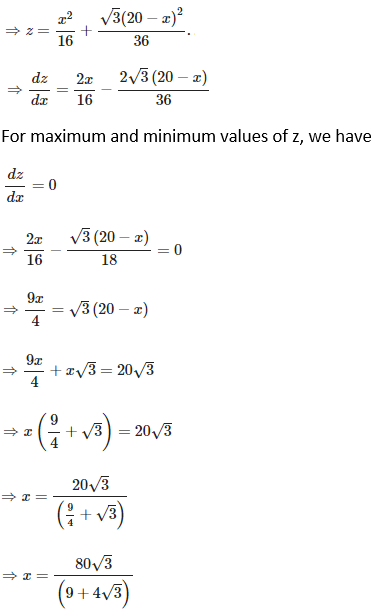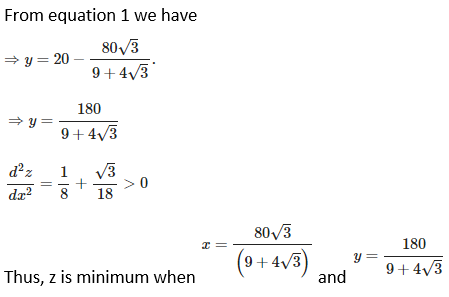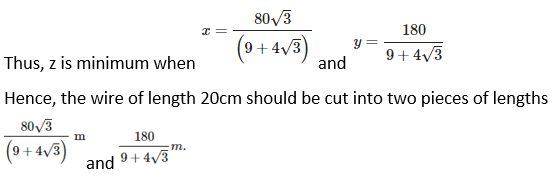Hence, the wire of length 20 m should be cut into two pieces of lengths9. Given the sum of the perimeters of a square and a circle, show that the sum of their areas is least when one side of the square is equal to diameter of the circle.

Solution:

Let us say the sum of the perimeter of the square and circumference of the circle be L

Given the sum of the perimeters of a square and a circle.

Assuming the side of the square = a and the radius of the circle = r

Then, L = 4a + 2πr ⇒ a = (L – 2πr)/4… (1)

Let the sum of the area of the square and circle be S

So, S = a2 + πr2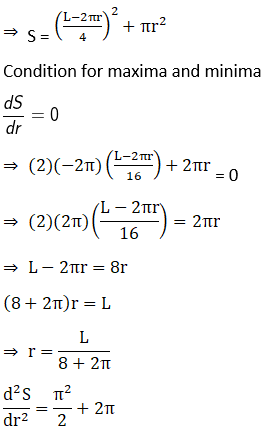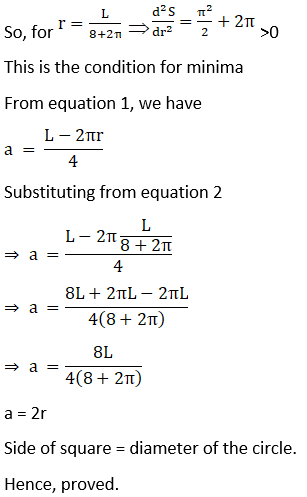10. Find the largest possible area of a right angled triangle whose hypotenuse is 5 cm long.

Solution: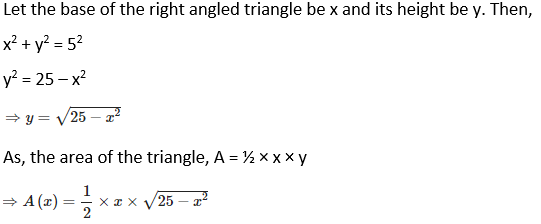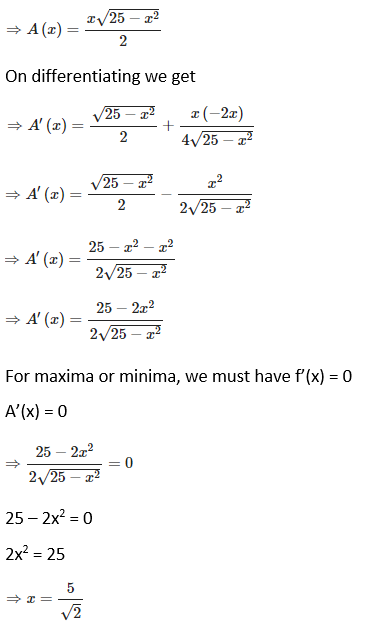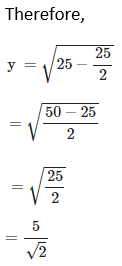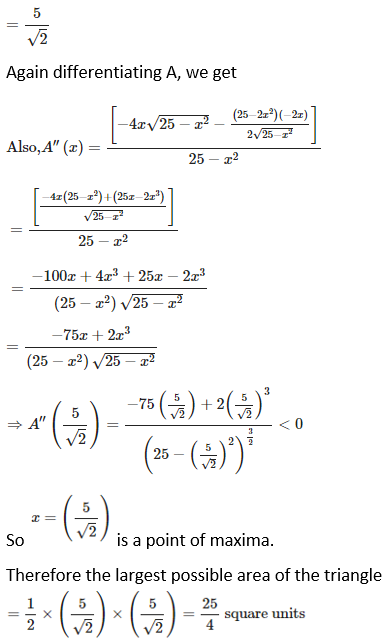11. Two sides of a triangle have lengths ‘a’ and ‘b’ and the angle between them is θ. What value of θ will maximize the area of the triangle? Find the maximum area of the triangle also.

Solution:

It is given that two sides of a triangle have lengths a and b, and the angle between them is θ.

Let the area of the triangle be A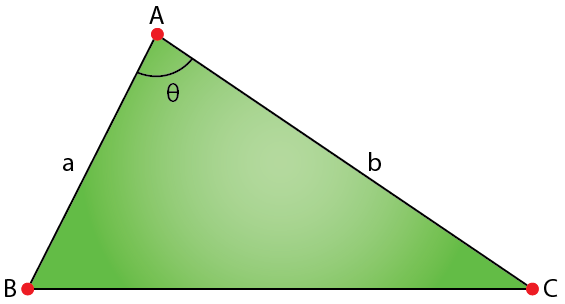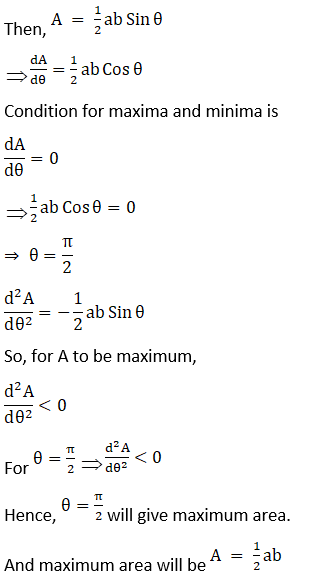12. A square piece of tin of side 18 cm is to be made into a box without top by cutting a square from each corner and folding up the flaps to form a box. What should be the side of the square to be cut off so that the volume of the box is maximum? Also, find this maximum volume

Solution: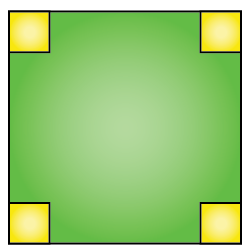Given side length of big square is 18 cm

Let the side length of each small square be a.

If by cutting a square from each corner and folding up the flaps we will get a cuboidal box with

Length, L = 18 – 2a

Breadth, B = 18 – 2a and

Height, H = a

Assuming, volume of box, V = LBH = a (18 – 2a)2

Condition for maxima and minima is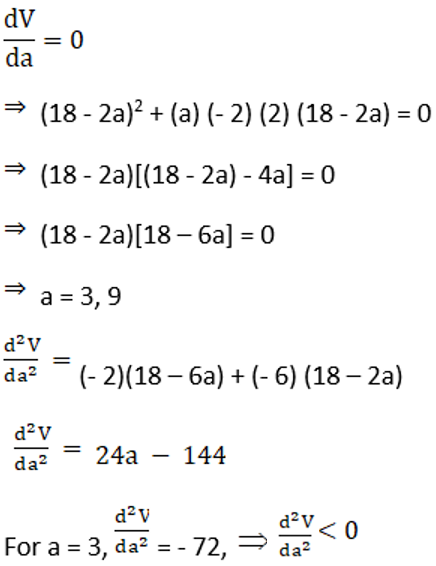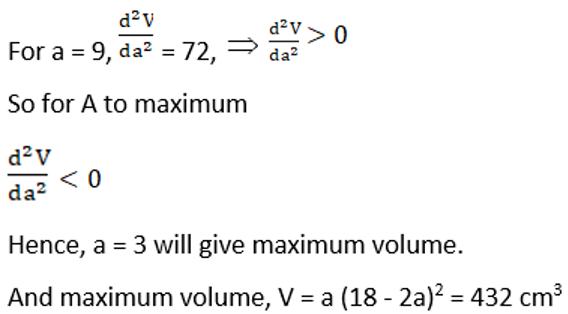13. A rectangular sheet of tin 45 cm by 24 cm is to be made into a box without top, by cutting off squares from each corners and folding up the flaps. What should be the side of the square to be cut off so that the volume of the box is maximum possible?

Solution: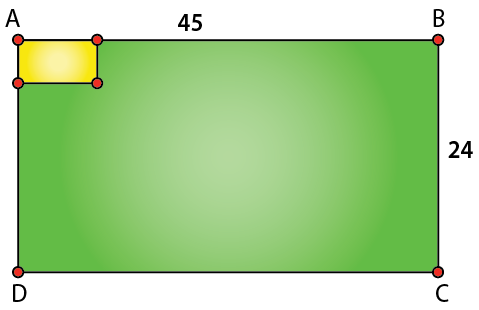Given the length of the rectangle sheet = 45 cm

Breath of rectangle sheet = 24 cm

Let the side length of each small square be a.

If by cutting a square from each corner and folding up the flaps we will get a cuboidal box with

Length, L = 45 – 2a

Breadth, B = 24 – 2a and

Height, H = a

Assuming, volume of box, V = LBH = (45 – 2a)(24 – 2a)(a)

Condition for maxima and minima is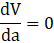(45 – 2a) (24 – 2a) + (- 2) (24 – 2a) (a) + (45 – 2a) (- 2)(a) = 0

4a2 – 138a + 1080 + 4a2 – 48a + 4a2 – 90a = 0

12a2 – 276a + 1080= 0

a2 – 23a + 90= 0

a = 5, 18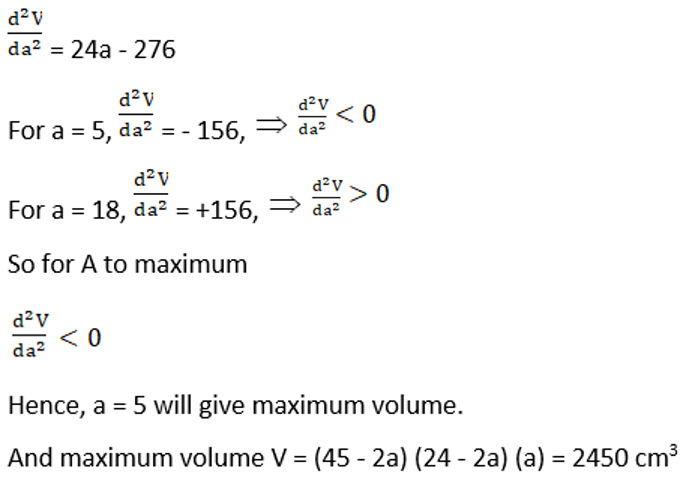14. A tank with rectangular base and rectangular sides, open at the top is to be constructed so that its depth is 2 m and volume is 8 m3. If building of tank cost Rs 70 per square metre for the base and Rs 45 per square metre for sides, what is the cost of least expensive tank?

Solution:

Let the length, breadth and height of the tank be l, b and h, respectively.

Also, assume the volume of the tank as V

h = 2 m (given)

V = 8 m3

l b h = 8

2lb = 8 (given)

lb = 4

b = 4/l … (1)

Cost for building base = Rs 70/m2

Cost for building sides = Rs 45/m2

Cost for building the tank, C = Cost for base + cost for sides

C = lb × 70 + 2(l + b) h × 45

C = l(4/l) × 70 + 2(l + 4/l) (2) × 45 [Using (1)]

= 280 + 180 (l + 4/l)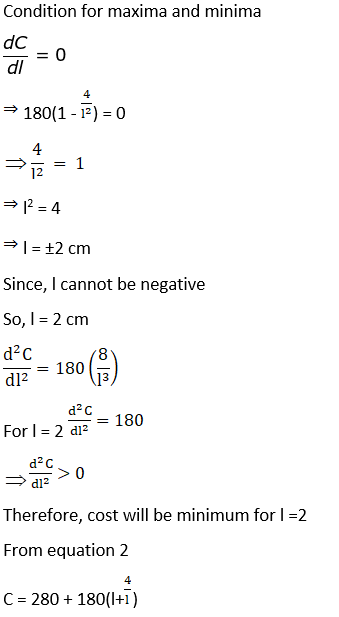C = Rs 1000

15. A window in the form of a rectangle is surmounted by a semi-circular opening. The total perimeter of the window is 10 m. Find the dimensions of the rectangular part of the window to admit maximum light through the whole opening.

Solution: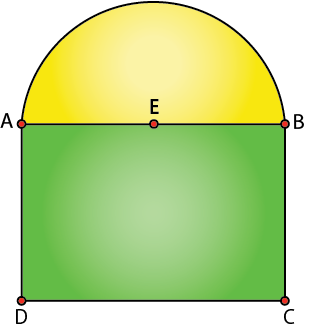Let the radius of the semicircle, length and breadth of the rectangle be r, x and y respectively

AE = r

AB = x = 2r (semicircle is mounted over rectangle) …1

Given the Perimeter of the window = 10 m

x + 2y + πr = 10

2r + 2y + πr = 10

2y = 10 – (π + 2).r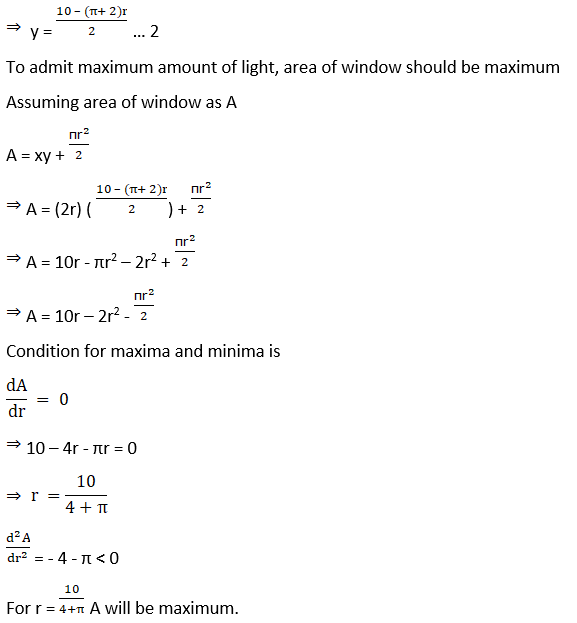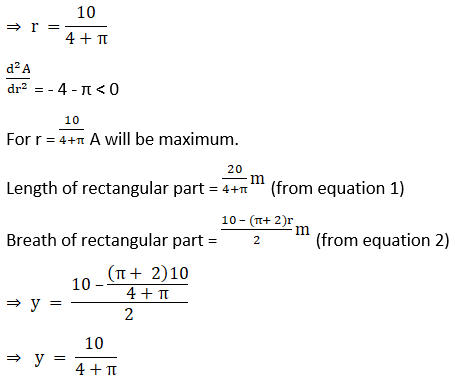16. A large window has the shape of a rectangle surmounted by an equilateral triangle. If the perimeter of the window is 12 metres find the dimensions of the rectangle that will produce the largest area of the window.

Solution:

Let the dimensions of the rectangle be x and y.

Therefore, the perimeter of window = x + y + x + x + y = 12

3x + 2y = 12

y = (12 – 3x)/2 …. (1)

Now,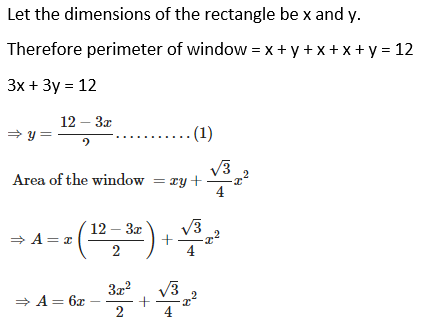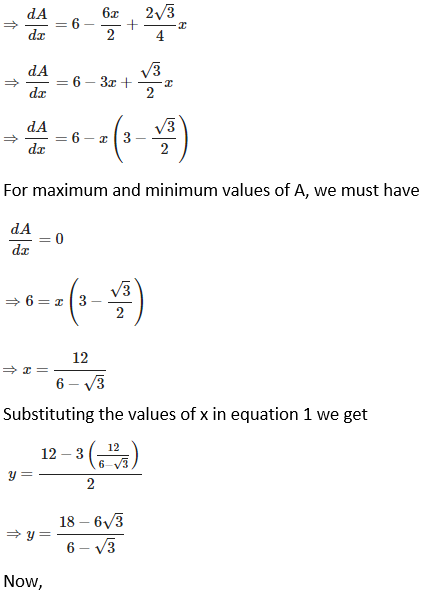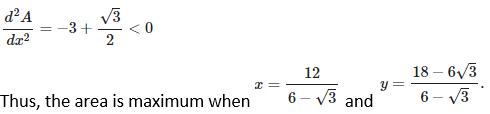17. Show that the height of the cylinder of maximum volume that can be inscribed in a sphere of radius R is 2R/√3.

Solution: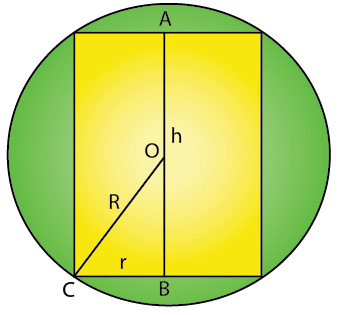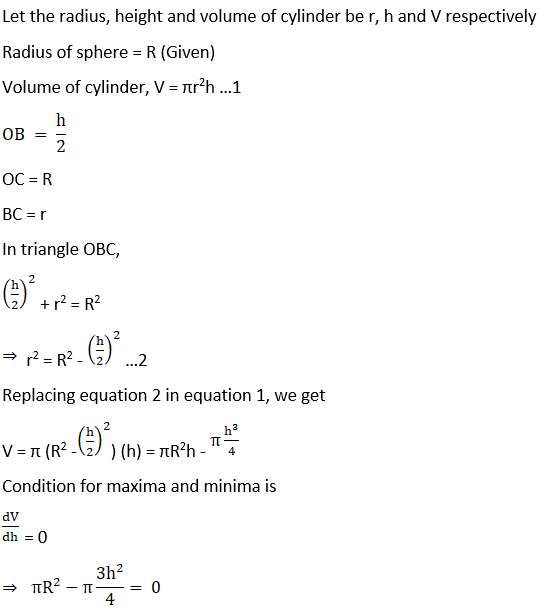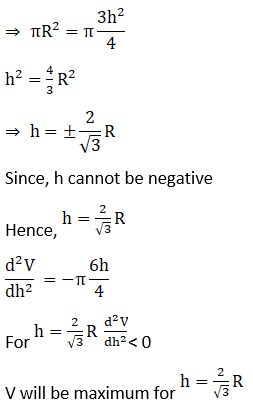18. A rectangle is inscribed in a semi-circle of radius r with one of its sides on diameter of semi-circle. Find the dimensions of the rectangle so that its area is maximum. Find also the area.

Solution: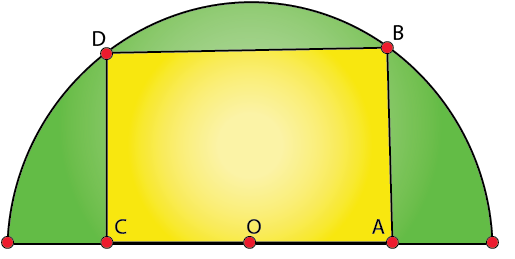Let the length and breadth of rectangle ABCD be 2x and y, respectively

The radius of semicircle = r (given)

In triangle OBA, where is the centre of the circle and mid-point of the side AC

r2 = x2 + y2 (Pythagoras theorem)

y2 = r2 – x2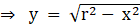… (1)

Let us say the area of the rectangle = A = xy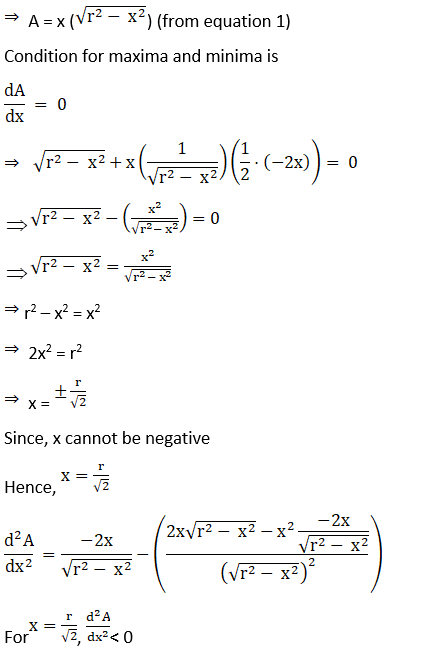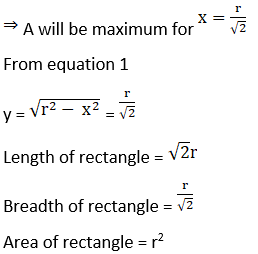[Since, l = 2x]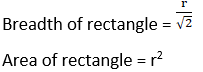19. Prove that a conical tent of given capacity will require the least amount of canvas when the height is √2 times the radius of the base.

Solution: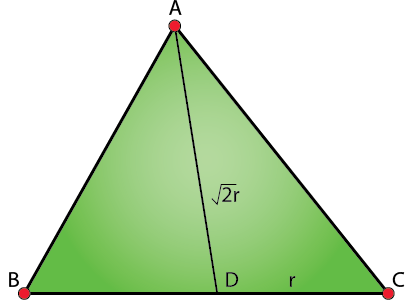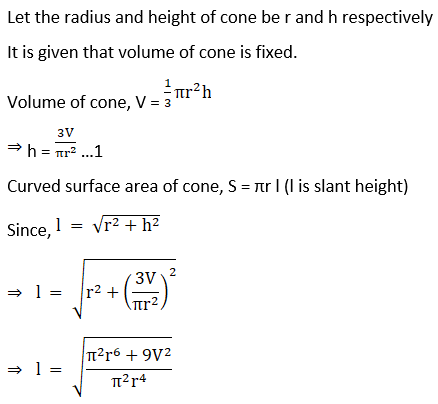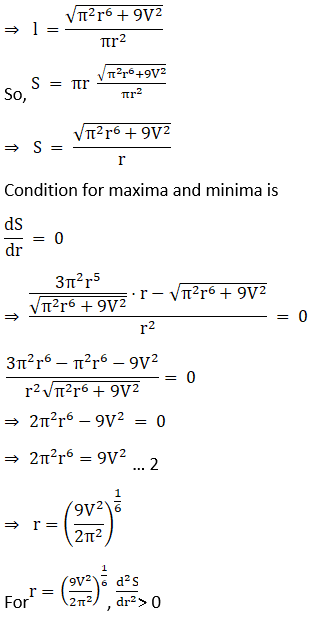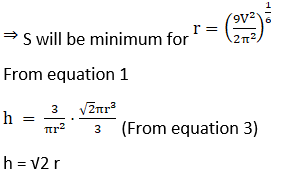20. Show that the cone of the greatest volume which can be inscribed in a given sphere has an altitude equal to 2/3 of the diameter of the sphere.

Solution: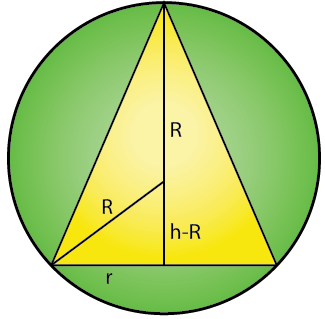Let the radius and height of the cone be r and h, respectively

The radius of the sphere = R

R2 = r2 + (h – R)2

R2 = r2 + h2 + R2 – 2hR

r2 = 2hR – h2 … (1)

Assuming the volume of the cone to be V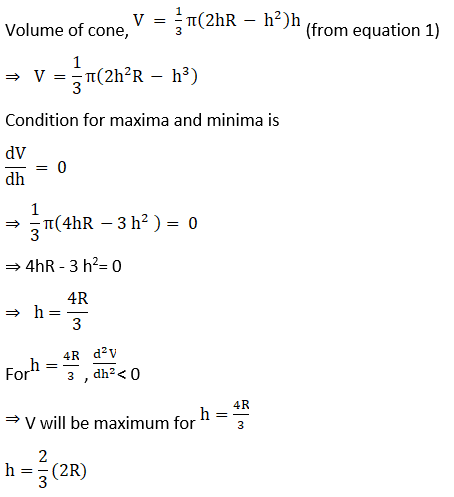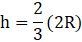21. Prove that the semi – vertical angle of the right circular cone of given volume and least curved surface is cot-1√2

Solution: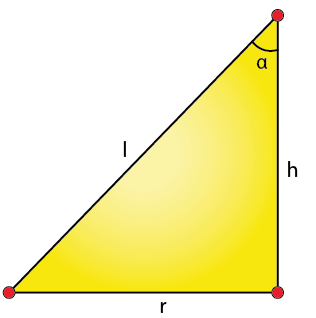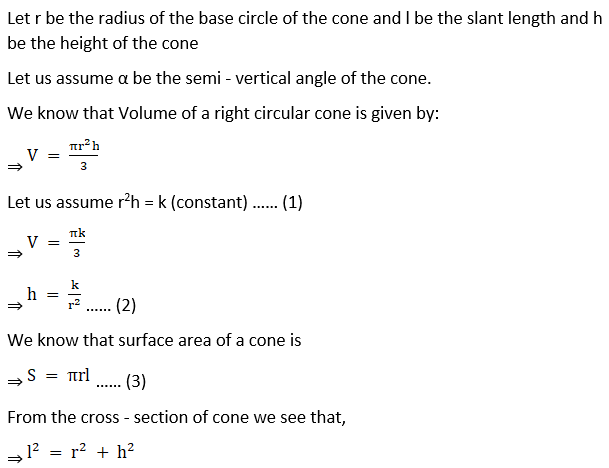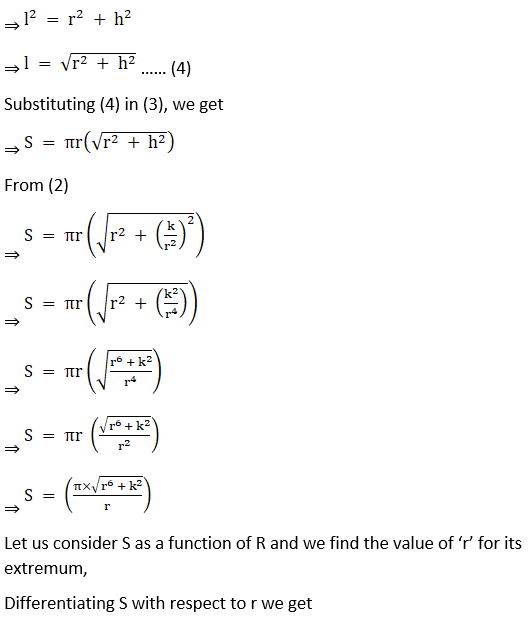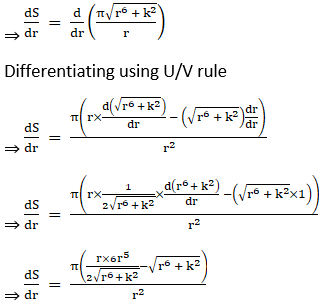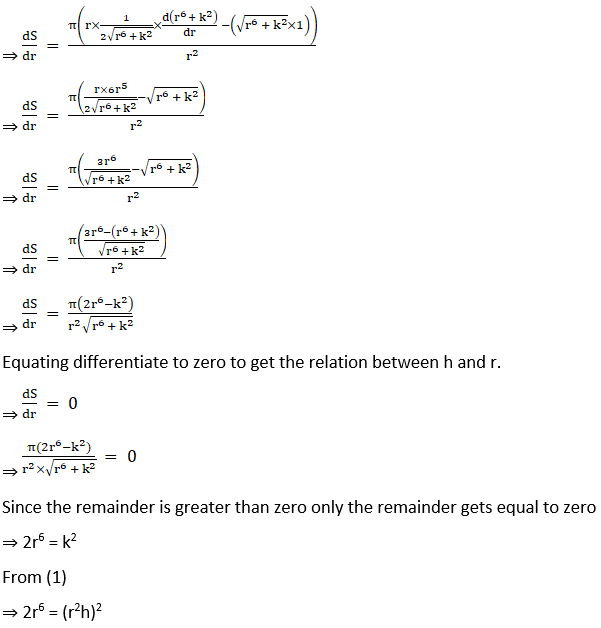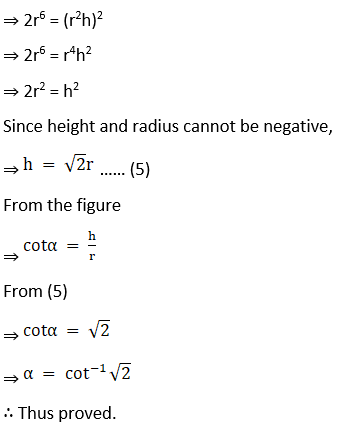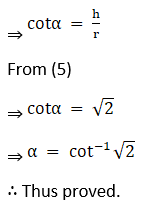22. An isosceles triangle of vertical angle 2θ is inscribed in a circle of radius a. Show that the area of the triangle is maximum when θ = π/6.

Solution: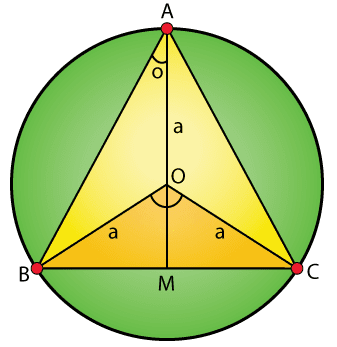Δ ABC is an isosceles triangle such that AB = AC.

The vertical angle BAC = 2θ

Triangle is inscribed in the circle with centre O and radius a.

Draw AM perpendicular to BC.

Since Δ ABC is an isosceles triangle, the circumcenter of the circle will lie on the perpendicular from A to BC.

Let O be the circumcenter.

BOC = 2 × 2θ = 4θ (Using central angle theorem)

COM = 2θ (Since, Δ OMB and Δ OMC are congruent triangles)

OA = OB = OC = a (radius of the circle)

In Δ OMC,

CM = asin2θ

OM = acos2θ

BC = 2CM (Perpendicular from the center bisects the chord)

BC = 2asin2θ

Height of Δ ABC = AM = AO + OM

AM = a + acos2θ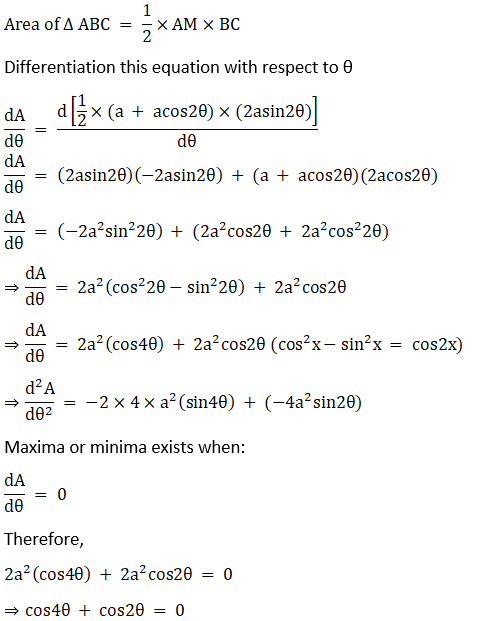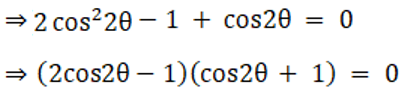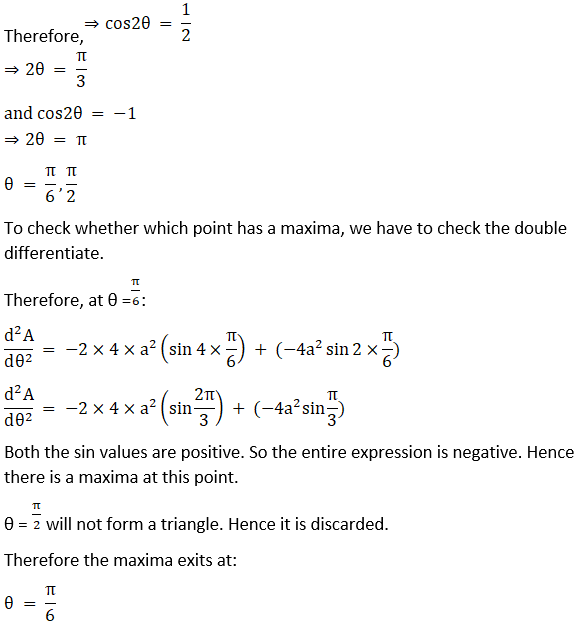23. Prove that the least perimeter of an isosceles triangle in which a circle of radius r can be inscribed is 6√3r.

Solution: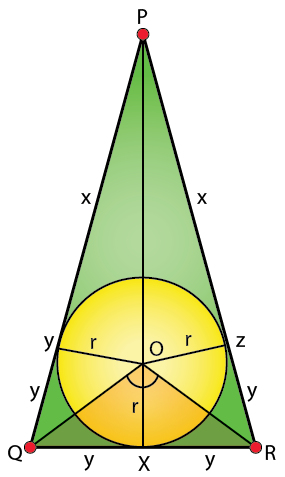QR at X and PR at Z.

OZ, OX, OY are perpendicular to the sides PR, QR, PQ.

Here PQR is an isosceles triangle with sides PQ = PR, and also from the figure,

⇒ PY = PZ = x

⇒ YQ = QX = XR = RZ = y

From the figure we can see that,

⇒ Area (ΔPQR) = Area (ΔPOR) + Area (ΔPOQ) + Area (ΔQOR)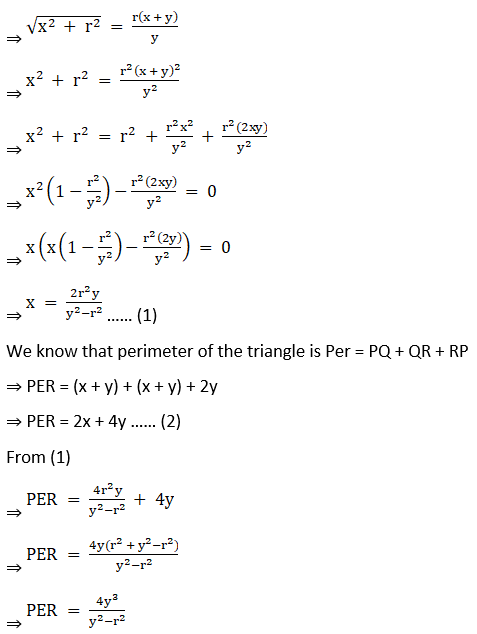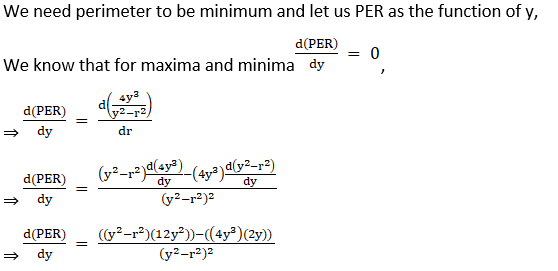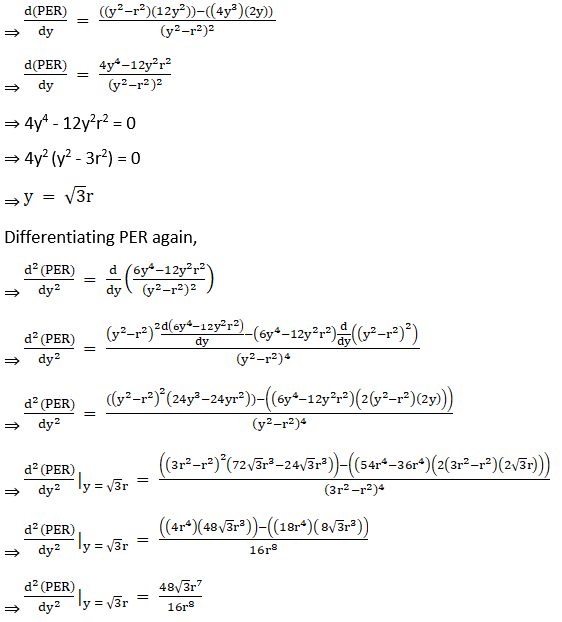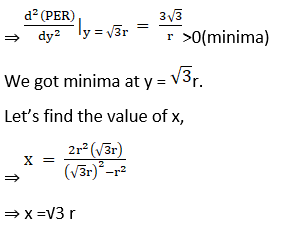⇒ PER = 2(√3r) + 4(√3r)

⇒ PER = 6√3r

∴ Thus proved